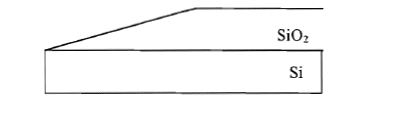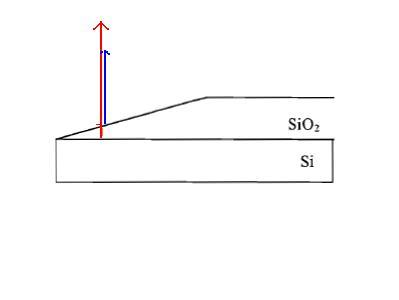# Interference in Thin Films

## Homework Statement

In order to decide the thickness of a SiO2 layer(n=1.5) on Si, a wedge is prepared as shown in the picture. A total of 7 DARK fringes was observed, when light of wavelength 589nm shines prependicularly on the wedge. It is known that light reflected from the Si/SiO2 surface would have a phase change of π rad.( I don't know why the image cannot appear after posting, it does appear in edit mode. Here is the link:
http://s3.postimg.org/cbvntdri9/physexam1q3.png)

i) Determine whether the fringe at the thinner end of the SiO2 wedge is bright or dark. Explain briefly. (2M)
ii) Calculate the thickness of the SiO2 layer. (3M)
iii) If the setup is illuminated by a light of shorter wavelength, what chages would you expect for the number of fringes observed? Explain briefly. (2M)
iv) If the setup is placed in water(n=1.33), what changes would you expect for the number of fringes observed? Explain your answer briefly. (2M)

## Homework Equations

For constructive interference, 2nt = (m+1/2)λ, m = 0, 1, 2......
For destructive interference, 2nt = mλ, m = 0, 1, 2......

## The Attempt at a Solution

i) Dark? Just guess. What should I consider?
ii) 2nt = mλ, m = 7
2(1.5)t = 7*(589*10^-9)
t = 1.96*10^-7m
iii) Just guess: Because 2nt = mλ, where 2nt is a constant. When λ decreases, m has to be increased. So the number of fringes observed increases.
iv) Same? But it doesn't seem correct......

Thank you!!

ehild
Homework Helper

## Homework Statement

In order to decide the thickness of a SiO2 layer(n=1.5) on Si, a wedge is prepared as shown in the picture. A total of 7 DARK fringes was observed, when light of wavelength 589nm shines prependicularly on the wedge. It is known that light reflected from the Si/SiO2 surface would have a phase change of π rad.( I don't know why the image cannot appear after posting, it does appear in edit mode. Here is the link: http://s3.postimg.org/cbvntdri9/physexam1q3.png

i) Determine whether the fringe at the thinner end of the SiO2 wedge is bright or dark. Explain briefly. (2M)
ii) Calculate the thickness of the SiO2 layer. (3M)
iii) If the setup is illuminated by a light of shorter wavelength, what chages would you expect for the number of fringes observed? Explain briefly. (2M)
iv) If the setup is placed in water(n=1.33), what changes would you expect for the number of fringes observed? Explain your answer briefly. (2M)

## Homework Equations

For constructive interference, 2nt = (m+1/2)λ, m = 0, 1, 2......
For destructive interference, 2nt = mλ, m = 0, 1, 2......

You observe reflection. Interference happens between the directly reflected ray (blue) and the one, entering into the layer and reflected back from the SiO2-Si interface (red)
For constructive interference, the phase difference between the blue and red rays should be integer times 2pi. What is the phase change of the directly reflected light?
What are the correct equations for constructive and destructive interference?

You observe reflection. Interference happens between the directly reflected ray (blue) and the one, entering into the layer and reflected back from the SiO2-Si interface (red)
For constructive interference, the phase difference between the blue and red rays should be integer times 2pi. What is the phase change of the directly reflected light?
What are the correct equations for constructive and destructive interference?

The equations are
"For constructive interference, path difference = mλ, m = 0, ±1, ±2......
For destructive interference, path difference = (m + 1/2)λ, m = 0, ±1, ±2......" ?

And both the red ray and the blue ray has undergone phase change?
Since SiO2's n=1.5> air's n =1, the blue ray has phase change of pi rad.
And it's given that the red ray has a phase change of pi rad.

ehild
Homework Helper
The equations are
"For constructive interference, path difference = mλ, m = 0, ±1, ±2......
For destructive interference, path difference = (m + 1/2)λ, m = 0, ±1, ±2......" ?

And both the red ray and the blue ray has undergone phase change?
Since SiO2's n=1.5> air's n =1, the blue ray has phase change of pi rad.
And it's given that the red ray has a phase change of pi rad.
They both undergo phase change at the boundary from where the reflect.

They both undergo phase change at the boundary from where the reflect.

But the path difference between they reflect changes continuously in part a)? How should I deal with this?

ehild
Homework Helper
You see a dark strip on the wedge where the thickness of SiO2 corresponds to the condition of destructive interference.The thickness depends on x, the horizontal distance from the edge and the angle of the incline - how?

You see a dark strip on the wedge where the thickness of SiO2 corresponds to the condition of destructive interference.The thickness depends on x, the horizontal distance from the edge and the angle of the incline - how?

tanθ=thickness/horizontal distance,
thickness=horizontal distance*tanθ?

ehild
Homework Helper
tanθ=thickness/horizontal distance,
thickness=horizontal distance*tanθ?
Yes. What should be the thickness at the first dark strip?

Yes. What should be the thickness at the first dark strip?

1/2λ=1/2*589=294.5nm?

ehild
Homework Helper
The wavelength 589 nm is given for air. You have to multiply the thickness by the refractive index. And the ray travels the thickness twice, once forward, then backward.

The wavelength 589 nm is given for air. You have to multiply the thickness by the refractive index. And the ray travels the thickness twice, once forward, then backward.
Oh yes. 2*1.5*thickness=1/2λ=294.5, thickness=294.5/3=589/6nm?

ehild
Homework Helper
Good. You see the first dark fringe where the thickness is 589/6 nm. Where do you see the seventh one?